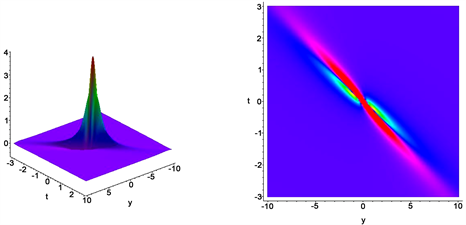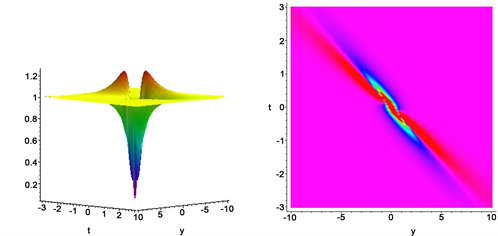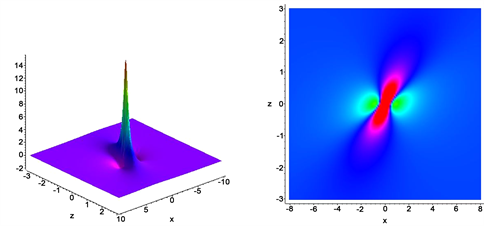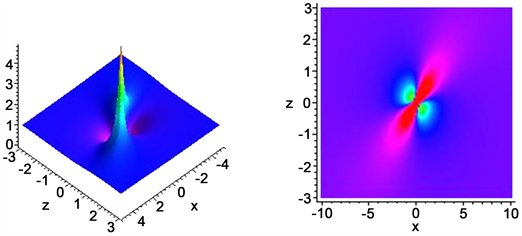# (3+1)维Mel’nikov方程的Lump解研究Lump Solutions to the (3+1)-Dimensional Mel’nikov Equation

• 全文下载: PDF(750KB)    PP.1058-1063   DOI: 10.12677/AAM.2019.86121
• 下载量: 131  浏览量: 228   科研立项经费支持

In this article, lump solutions of the (3+1)-dimensional Mel’nikov are obtained via the Hirota bi-linear method and symbolic computation with Maple. A class of lump solutions rationally localized in all directions in the space is obtained. And we propose the conditions for the analyticity and ra-tional localization of the lump solutions. By selecting special value of parameters involved, the dynamic characteristics of the solutions are illustrated.

1. 引言

$\left\{\begin{array}{l}{\left({u}_{t}+6u{u}_{x}+{u}_{xxx}+8{|\varphi |}_{x}^{2}\right)}_{x}-{u}_{yy}+{u}_{zz}=0,\\ i{\varphi }_{y}=2{\varphi }_{xx}+2u\varphi ,\\ i{\varphi }_{z}={\varphi }_{xx}+u\varphi ,\end{array}$ (1)

2. Mel’nikov的Lump解

$\left\{\begin{array}{l}u=2{\left(\mathrm{ln}f\right)}_{xx},\\ \varphi =\frac{g}{f},\end{array}$ (2)

$\left\{\begin{array}{l}\left({D}_{x}{D}_{t}+{D}_{x}^{4}-{D}_{y}^{2}+{D}_{z}^{2}\right)f\cdot f+8\left(g\stackrel{¯}{g}-{f}^{2}\right)=0,\\ \left(i{D}_{y}-2{D}_{x}^{2}\right)g\cdot f=0,\\ \left(i{D}_{z}-{D}_{x}^{2}\right)g\cdot f=0.\end{array}$ (3)

${D}_{x}^{m}{D}_{t}^{n}\left(a,b\right)={{\left(\frac{\partial }{\partial x}-\frac{\partial }{\partial {x}^{\prime }}\right)}^{m}{\left(\frac{\partial }{\partial t}-\frac{\partial }{\partial {t}^{\prime }}\right)}^{n}a\left(x,t\right)b\left({x}^{\prime },{t}^{\prime }\right)|}_{{x}^{\prime }=x,{t}^{\prime }=t}$ (4)

$\left\{\begin{array}{l}f=1+{s}^{2}+{h}^{2},\\ {g}_{R}={b}_{0}+{b}_{1}s+{b}_{2}h+{b}_{3}{s}^{2}+{b}_{4}{h}^{2},\\ {g}_{I}={c}_{0}+{c}_{1}s+{c}_{2}h+{c}_{3}{s}^{2}+{c}_{4}{h}^{2},\end{array}$ (5)

$\left\{\begin{array}{l}s={a}_{1}x+{a}_{2}y+{a}_{3}z+{a}_{4}t+{a}_{5},\\ h={a}_{6}x+{a}_{7}y+{a}_{8}z+{a}_{9}t+{a}_{10},\end{array}$ (6)

$\begin{array}{l}\left\{{a}_{2}=2{a}_{3},{a}_{4}=\frac{\left({a}_{3}^{2}-{a}_{1}^{4}\right)\left[3{\left({a}_{1}^{4}+{a}_{3}^{2}\right)}^{2}+16{a}_{1}^{4}\right]}{{a}_{1}{\left({a}_{1}^{4}+{a}_{3}^{2}\right)}^{2}},{a}_{6}=0,{a}_{7}=2{a}_{1}^{2},{a}_{8}=\frac{1}{2}{a}_{7},{a}_{9}=\frac{2{a}_{1}{a}_{3}\left[3{\left({a}_{1}^{4}+{a}_{3}^{2}\right)}^{2}-16{a}_{1}^{4}\right]}{{\left({a}_{1}^{4}+{a}_{3}^{2}\right)}^{2}},\\ {b}_{0}=\frac{{b}_{3}\left({a}_{3}^{2}-3{a}_{1}^{4}\right)}{{a}_{1}^{4}+{a}_{3}^{2}},{b}_{1}=k{c}_{1},{b}_{2}=k{c}_{2},{b}_{4}={b}_{3},{c}_{0}=-k{b}_{0},{c}_{1}=-\frac{4{a}_{3}{b}_{3}{a}_{1}^{2}}{{a}_{1}^{4}+{a}_{3}^{2}},{c}_{2}=\frac{4{a}_{1}^{4}{b}_{3}}{{a}_{1}^{4}+{a}_{3}^{2}},{c}_{3}=-k{b}_{3},{c}_{4}={c}_{3}\right\}\end{array}$ (7)

${b}_{3}^{2}\left(1+{k}^{2}\right)=1$ (8)

$\left\{\begin{array}{l}u=\frac{4{a}_{1}^{2}\left(1-{s}^{2}+{h}^{2}\right)}{{\left(1+{s}^{2}+{h}^{2}\right)}^{2}},\\ \varphi =\frac{{g}_{R}+i{g}_{I}}{1+{s}^{2}+{h}^{2}},\end{array}$ (9)

$\begin{array}{l}{g}_{R}=\frac{{b}_{3}\left({a}_{3}^{2}-3{a}_{1}^{4}\right)}{{a}_{1}^{4}\text{+}{a}_{3}^{2}}-k\frac{4{a}_{3}{b}_{3}{a}_{1}^{2}}{{a}_{1}^{4}\text{+}{a}_{3}^{2}}s+k\frac{4{a}_{1}^{4}{b}_{3}}{{a}_{1}^{4}\text{+}{a}_{3}^{2}}h+{b}_{3}\left({s}^{2}+{h}^{2}\right),\\ {g}_{I}=-k\frac{{b}_{3}\left({a}_{3}^{2}-3{a}_{1}^{4}\right)}{{a}_{1}^{4}\text{+}{a}_{3}^{2}}-\frac{4{a}_{3}{b}_{3}{a}_{1}^{2}}{{a}_{1}^{4}\text{+}{a}_{3}^{2}}s+\frac{4{a}_{1}^{4}{b}_{3}}{{a}_{1}^{4}\text{+}{a}_{3}^{2}}h-k{b}_{3}\left({s}^{2}+{h}^{2}\right),\end{array}$ (10)

$\begin{array}{l}s={a}_{1}x+2{a}_{3}y+{a}_{3}z+\frac{\left({a}_{3}^{2}-{a}_{1}^{4}\right)\left[3{\left({a}_{1}^{4}+{a}_{3}^{2}\right)}^{2}\text{+}16{a}_{1}^{4}\right]}{{a}_{1}{\left({a}_{1}^{4}+{a}_{3}^{2}\right)}^{2}}t+{a}_{5},\\ h=2{a}_{1}^{2}y+{a}_{1}^{2}z+\frac{2{a}_{1}{a}_{3}\left[3{\left({a}_{1}^{4}+{a}_{3}^{2}\right)}^{2}-16{a}_{1}^{4}\right]}{{\left({a}_{1}^{4}+{a}_{3}^{2}\right)}^{2}}t+{a}_{10}.\end{array}$ (11)Figure 1. Plots of lump solution for u with ${a}_{1}=1,\text{\hspace{0.17em}}{a}_{3}=2,\text{\hspace{0.17em}}{b}_{3}=-0.8,\text{\hspace{0.17em}}{a}_{5}={a}_{10}=0,\text{\hspace{0.17em}}k=0.75$ when $x=0,\text{\hspace{0.17em}}z=0$Figure 2. Plots of lump solution for ${|\varphi |}^{2}$ with ${a}_{1}=1,\text{\hspace{0.17em}}{a}_{3}=2,\text{\hspace{0.17em}}{b}_{3}=-0.8,\text{\hspace{0.17em}}{a}_{5}={a}_{10}=0,\text{\hspace{0.17em}}k=0.75$ when $x=0,\text{\hspace{0.17em}}z=0$Figure 3. Plots of lump solution for u with ${a}_{1}=2,\text{\hspace{0.17em}}{a}_{3}=-2,\text{\hspace{0.17em}}{b}_{3}=0.8,\text{\hspace{0.17em}}{a}_{5}={a}_{10}=0,\text{\hspace{0.17em}}k=0.75$ when $y=0,\text{\hspace{0.17em}}t=0$Figure 4. Plots of lump solution for ${|\varphi |}^{2}$ with ${a}_{1}=2,\text{\hspace{0.17em}}{a}_{3}=-2,\text{\hspace{0.17em}}{b}_{3}=0.8,\text{\hspace{0.17em}}{a}_{5}={a}_{10}=0,\text{\hspace{0.17em}}k=0.75$ when $y=0,\text{\hspace{0.17em}}t=0$

3. 结论

NOTES

*通讯作者。

  Mel’Nikov, V.K. (1983) On Equations for Wave Interactions. Letters in Mathematical Physics, 7, 129-136. https://doi.org/10.1007/BF00419931  Lin, R.-L., Liu, X.-J. and Zeng, Y.-B. (2014) The KP Hierarchy with Self-Consistent Sources: Construction, Wronskian Solutions and Bilinear Identities. Journal of Physics: Conference Series, 538, Article No. 012014. https://doi.org/10.1088/1742-6596/538/1/012014  Lin, R.-L., Zeng, Y.-B. and Ma, W.-X. (2001) Solving the KdV Hierarchy with Self-Consistent Sources by Inverse Scattering Method. Physica A: Statistical Mechanics and Its Applications, 291, 287-298. https://doi.org/10.1016/S0378-4371(00)00519-7  Xiao, T. and Zeng, Y.-B. (2004) Generalized Darboux Transformations for the KP Equation with Self-Consistent Sources. Journal of Physics A: Mathematical and General, 37, 7143-7162. https://doi.org/10.1088/0305-4470/37/28/006  Wang, H.-Y. and Hu, X.-B. (2001) A Hybrid Type of Soliton Equations with Self-Consistent Sources: KP and Toda Cases. Journal of Mathematical Analysis and Applications, 376, 393-406. https://doi.org/10.1016/j.jmaa.2010.12.010  Zeng, Y.-B., Ma, W.-X. and Lin, R.-L. (2001) Integra-tion of the Soliton Hierarchy with Self-Consistent Sources. Journal of Mathematical Physics, 41, 5453-5489. https://doi.org/10.1063/1.533420  Deng, S.-F., Chen, D.-Y. and Zhang, D.-J. (2003) The Multisoliton Solu-tions of the KP Equation with Self-Consistent Sources. Journal of the Physical Society of Japan, 72, 2184-2192. https://doi.org/10.1143/JPSJ.72.2184  Hu, X.-B. and Wang, H.-Y. (2006) Construction of dKP and BKP Equations with Self Consistent Sources. Inverse Problems, 22, 1903-1920. https://doi.org/10.1088/0266-5611/22/5/022  Yao, Y.-Q. and Zeng, Y.-B. (2008) Integrable Rosochatius De-formations of Higher-Order Constrained Flows and the Soliton Hierarchy with Self-Consistent Sources. Journal of Physics A: Mathematical and Theoretical, 41, Article No. 295205. https://doi.org/10.1088/1751-8113/41/29/295205  Zhu, J.-Y. and Geng, X.-G. (2012) A Hierarchy of Coupled Evolution Equations with Self-Consistent Sources and the Dressing Method. Journal of Physics A: Mathematica and Theoretical, 46, Article No. 035204. https://doi.org/10.1088/1751-8113/46/3/035204  Wang, H. and Xia, T.-C. (2013) Conservation Laws and Self-Consistent Sources for a Super KN Hierarchy. Applied Mathematics and Computation, 219, 5458-5464. https://doi.org/10.1016/j.amc.2012.11.042  Ma, W.-X. (2015) Lump Solutions to the Kadomtsev-Petviashvili Equation. Physics Letters A, 379, 1975-1978. https://doi.org/10.1016/j.physleta.2015.06.061  Yang, J.-Y. and Ma, W.-X. (2017) Abundant Interaction So-lutions of the KP Equation. Nonlinear Dynamics, 89, 1539-1544. https://doi.org/10.1007/s11071-017-3533-y  Zhao, H.-Q. and Ma, W.-X. (2017) Mixed Lump-Kink Solutions to the KP Equation. Computers & Mathematics with Applications, 74, 1399-1405. https://doi.org/10.1016/j.camwa.2017.06.034  Yang, J.-Y. and Ma, W.-X. (2016) Lump Solutions of the BKP Equation by Symbolic Computation. International Journal of Modern Physics B, 30, Article No. 1640028. https://doi.org/10.1142/S0217979216400282  Zhang, J.-B. and Ma, W.-X. (2017) Mixed Lump-Kink Solu-tions to the BKP Equation. Computers & Mathematics with Applications, 74, 591-596. https://doi.org/10.1016/j.camwa.2017.05.010  Ma, W.-X., Yong, X.-L. and Zhang, H.-Q. (2018) Diversity of Interaction Solutions to the (2+1)-Dimensional Ito Equation. Computers & Mathematics with Applications, 75, 289-295. https://doi.org/10.1016/j.camwa.2017.09.013  Yong, X.-L., Ma, W.-X., Huang, Y.-H. and Liu, Y. (2018) Lump Solutions to the Kadomtsev-Petviashvili I Equation with a Self-Consistent Source. Computers and Mathematics with Applications, 75, 3414-3419. https://doi.org/10.1016/j.camwa.2018.02.007  Ablowitz, M.J. and Satsuma, J. (1978) Solitons and Rational Solutions of Nonlinear Evolution Equations. Journal of Mathematical Physics, 19, 2180-2186. https://doi.org/10.1063/1.523550  Kaup, D.J. (1981) The Lump Solutions and the Backlund Transformation for the Three-Dimensional Three-Wave Resonant Interaction. Journal of Mathematical Physics, 22, 1176-1181. https://doi.org/10.1063/1.525042  Gilson, C.R. and Nimmo, J.J.C. (1990) Lump Solutions of the BKP Equa-tion. Physics Letters A, 147, 472-476. https://doi.org/10.1016/0375-9601(90)90609-R  Imai, K. (1997) Dromion and Lump Solutions of the Ishimori-I Equation. Progress of Theoretical Physics, 98, 1013-1023. https://doi.org/10.1143/PTP.98.1013  Zhang, X. and Chen, Y. (2017) Rogue Wave and a Pair of Resonance Stripe Solitons to a Reduced (3+1)-Dimensional Jimbo—Miwa Equation. Communications in Nonlinear Science and Numerical Simulation, 52, 24-31. https://doi.org/10.1016/j.cnsns.2017.03.021  Pu, J.-C. and Hu, H.-C. (2018) Mixed Lump—Soliton Solutions of the (3+1)-Dimensional Soliton Equation. Applied Mathematics Letters, 85, 77-81. https://doi.org/10.1016/j.aml.2018.05.017  Foroutan, M., Manafian, J. and Ranjbaran, A. (2018) Lump Solu-tion and Its Interaction to (3+1)-D Potential-YTSF Equation. Nonlinear Dynamics, 92, 2077-2092. https://doi.org/10.1007/s11071-018-4182-5  Zhang, Y., Liu, Y.-P. and Tang, X.-Y. (2018) M-Lump and In-teractive Solutions to a (3+1)-Dimensional Nonlinear system. Nonlinear Dynamics, 93, 2533-2541. https://doi.org/10.1007/s11071-018-4340-9  Zhang, J.-G. and Zhang, Y.-F. (2018) Construction of Lump Soliton and Mixed Lump Stripe Solutions of (3+1)- Dimensional Soliton Equation. Results in Physics, 10, 94-98. https://doi.org/10.1016/j.rinp.2018.05.022  Ma, W.-X. (2016) Lump-Type Solutions to the (3+1)-Dimensional Jimbo—Miwa Equation. International Journal of Nonlinear Sciences and Numerical Simulation, 17, 355-359. https://doi.org/10.1515/ijnsns-2015-0050  Yang, J.-Y. and Ma, W.-X. (2017) Abundant Lump-Type Solutions of the Jimbo—Miwa Equation in (3+1)-Dimensions. Computers and Mathematics with Applications, 73, 220-225. https://doi.org/10.1016/j.camwa.2016.11.007  Batwa, S. and Ma, W.-X. (2018) A Study of Lump-Type and Interaction Solutions to a (3+1)-Dimensional Jimbo—Miwa-Like Equation. Computers and Mathematics with Applications, 76, 1576-1582. https://doi.org/10.1016/j.camwa.2018.07.008  Wang, H.-Y. and Wen, D.-H. (2012) A (3+1)-Dimensional KP Equation with Self-Consistent Source. Journal of Zhengzhou University, 44, 6-10.  Hirota, R. (2004) The Direct Method in Soliton Theory. Cambridge University Press, New York. https://doi.org/10.1017/CBO9780511543043